Problem C1.2. Ringleb Problem

Overview

This problem tests the spatial accuracy of high-order methods. The flow is transonic and smooth. The geometry is also smooth, and high-order curved boundary representation appears to be critical. The exact solution is known via ‘hodograph transformation’ .

Governing Equations

The governing equations are the 2D Euler equations with.

Geometry

Let k be a streamline parameter, i.e., k = constant on each streamline. The two stream lines for the two wall boundaries are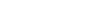for the inner wall, andfor the outer wall. Let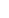be the velocity magnitude. For each fixed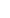,, the variablevaries between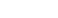and. For each, define the speed of sound, density, pressure, and a quantity denoted byby(1)

For each pair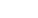, set(2)

 (a) Inflow

 (b) Outflow

 (d) Outer wall

 (c) Inner wall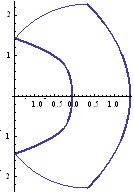Fig. 1.2  Ringleb geometry; thick curves: walls; thin curves: inflow and outflow boundaries.

With again,, and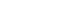, the four boundaries are: (a) inflow,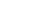,, and; (b) outflow,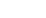,, and; (c) inner wall,and; and outer wall,and. See Fig. 1.

Exact Solution

The exact solution is given by (1) and (2). The flow is irrotational and isentropic. It reaches a supersonic speed of Mach number 1.5 at locationof the inner wall. The sonic line for the problem is shown in Fig. 2. Entropy should be a constant in the flow field.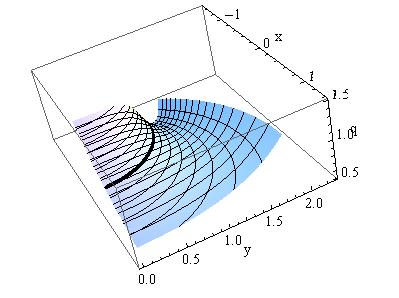Fig. 2. Plot of velocity magnitudeand streamlines for the region.

Requirements

1. Start the simulation from the exact solution, and monitor convergence using the L2 norm of the density residual. Compute the work units required to achieve steady state.

2. Similar to the smooth bump problem, use the non-dimensional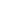entropy errors as accuracy indicator. Perform the calculations for at least three different meshes (quadruple the number of cells each time); use methods with different p to assess the performance of schemes of various orders.

3. To assess the efficiency of the spatial discretization, calculate the residual (right hand side) on the densest mesh 1000 times (equivalent to 1000 Euler forward time steps). Provides the results in work units.

4. Submit three sets of data to the workshop contact (above)

o   Errors vs mesh sizes for different p

o   Errors and work units for different h (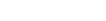) and p

o   Work units to complete the residual evaluation 1000 times for various  p.

References

 G. Chiocchia, Exact solutions to transonic and supersonic flows. Technical Report AR-211, AGARD, 1985.